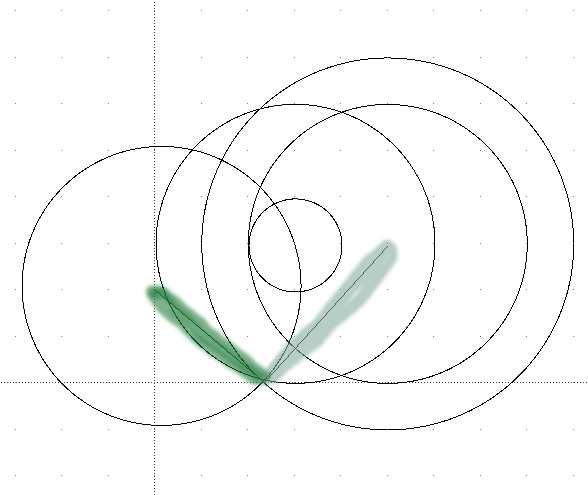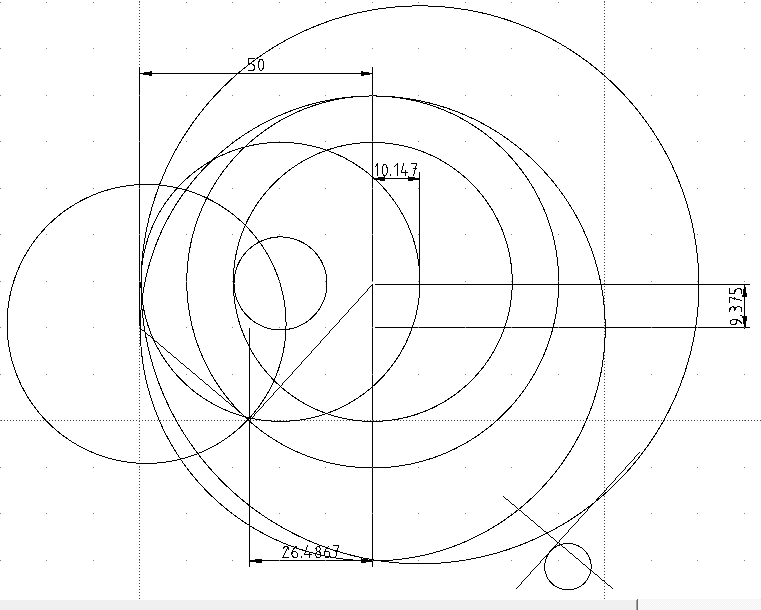_______ 20090328 recorded As we learned from the “ladder in the alley” problem, we have a graphical solution to the problem. So other than solving similar types of problems, there is a direct relationship to solving vectors! Of course by simple inspection, we could draw an encompassing circle around the elements of a vector. This is plain common sense. But what if we were dealing with multiple elements such as a dynamics problem involving relative velocity. Would it then be possible to find many frames of reference by doing only the vector addition of one frame? We will see but first we must draw a graphical representation that must work with most if not all vectors. Click here to review dynamics problem about relative velocity. Click here for DXF file of spiral. It is important to note that the reason such an easy, systematic way of solving this relative velocity problems is due to changing angular velocity. That is, the velocity apposed to a member that is rotating relative to a know velocity, can change. The angular velocity is going to change as the length of the circle changes. However we may still find something useful by using circles to explain vectors. I am not exactly sure how this graphical relationship will look like, but I will start with a few ideas I’ve been working on. First, picture a spiral of 2 vectors that are aligned. (Aligned meaning they have their maximum and minium lengths occur at the same angle.) If we place 2 circle representing the maximum length of each vector inside one large circle that is the sum of the maximum length of the 2 vectors, we now have a picture of the vector addition. That is, at least for that one frame.So with each different vector a circle representing its magnitude would be added to the previous circle. For the “ladder in the alley” problem a simple geometric construction solved the fact that the vectors crossed. The construction turned two crossing lengths into a tip to tail vector. That is why the construction is useful.That pattern of the spiral may also prove to be useful. My theory is the length of the individual loops form in the geometry from their tangents, are equal. And equal the total length of the circle. This could be proved by comparing the loops to the original vector circle. So back to the circles versus vectors, we can easily add circle to circle for how many members of the vector problem we have.I know this write-up is basically stating the obvious. That is, what can be seen by anyone with any knowledge of vectors. Again the real difficulty in relative velocity problems is that the velocity is given at that instant. Any knowledge of changing angles is not enough to solve the problem. I just have a hunch about this problem. There may be something there. The question is “can it be turned into a useful, logical, and reproducible solution?” May the Creative Force be with You